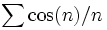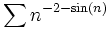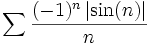# Landen's Legendary Limits

• Do the following limits exist? landen found these problems fairly hard. The second problem was especially tough. The third problem involves the famous dioid-landen constant.
•$\sum \cos(n)/n$ solution (http://www.efnet-math.org/math_tech/ToughTrig.html)
•$\sum n^{-2-\sin(n)}$ solution (http://www.efnet-math.org/math_tech/series5.pdf)
•$\sum \frac{(-1)^n \left | \sin(n) \right |}{n}$solution (http://www.efnet-math.org/math_tech/tseries3.pdf)
• Here is a discussion of two nested radicals from Ramanujan (http://www.efnet-math.org/public_html/math_tech/RamanujanRadicals.pdf)